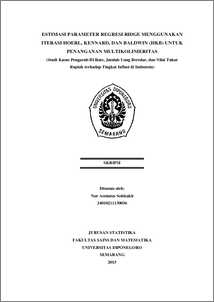# ESTIMASI PARAMETER REGRESI RIDGE MENGGUNAKAN ITERASI HOERL, KENNARD, DAN BALDWIN (HKB) UNTUK PENANGANAN MULTIKOLINIERITAS (Studi Kasus Pengaruh BI Rate, Jumlah Uang Beredar, dan Nilai Tukar Rupiah terhadap Tingkat Inflasi di Indonesia)

Solekakh, Nur Aeniatus (2015) ESTIMASI PARAMETER REGRESI RIDGE MENGGUNAKAN ITERASI HOERL, KENNARD, DAN BALDWIN (HKB) UNTUK PENANGANAN MULTIKOLINIERITAS (Studi Kasus Pengaruh BI Rate, Jumlah Uang Beredar, dan Nilai Tukar Rupiah terhadap Tingkat Inflasi di Indonesia). Undergraduate thesis, Fakultas Sains dan Matematika, Undip.Preview
PDF
1020Kb

## Abstract

Regression analysis is statistical method used to analyze the dependence of respond variables to predictor variable. In multiple linear regression analysis, there are assumptions that must be met, they are normality, homoscedasticity, absence of multicollinearity, and absence of autocorrelation. One of assumption frequently encountered is multicollinearity. If multicollineraity is exist between predictor variables, then regression analysis with ordinary least square is no longer used. Ridge regression is regression method used to handle multicollinearity. The ridge estimator involves adding biasing constant (k) to each diagonal element of X’X. Biasing constant (k) is determined by Hoerl, Kennard, and Baldwin (HKB) iteration method. This regression can be applied to inflation rate in Indonesia data and the factors that influence, they are BI rate, money supply, and exchange rate. Ridge regression analysis, the VIF (Variance Inflation Factor) values for each predictor variables BI rate, money supply, and exchange rate are 1.6637, 3.2712, and 4.3309. Since VIF values are not exceed to 10, then there is no multicollinearity in ridge egression model. Keywords: Inflation, Multikollinearity, Ridge Regression, HKB Iteration, VIF

Item Type: Thesis (Undergraduate) H Social Sciences > HA Statistics Faculty of Science and Mathematics > Department of Statistics 55123 Mr Hasbi Yasin 02 Aug 2017 09:31 02 Aug 2017 09:31

Repository Staff Only: item control page